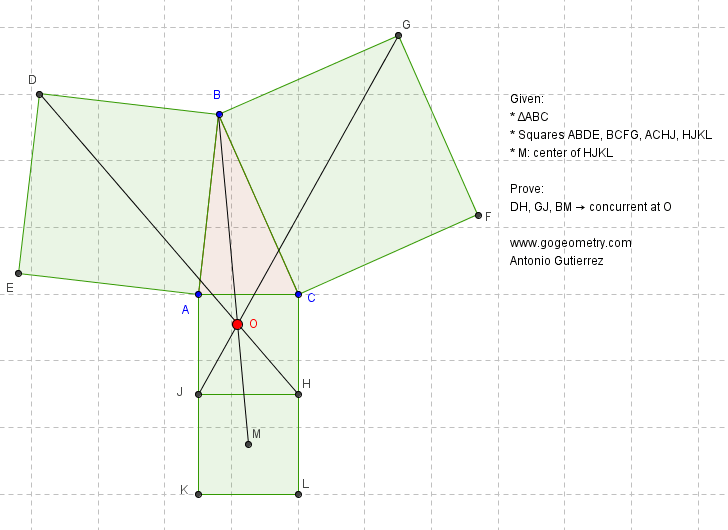# Static Diagram Geometry Problem 902: Triangle, Four Squares, Center, Concurrent Lines. Levels: School, College, Mathematics Education

< PREVIOUS PROBLEM  |  NEXT PROBLEM >Given a triangle ABC (see the figure above) with the squares ABDE, BCFG, ACHJ, and HJKL. If M is the center of square HJKL, prove that lines DH, GJ, and BM are concurrent at O.
See the dynamic diagram of problem 902

Home | SearchGeometry | Problems | All Problems | Open Problems | Visual Index | 10 Problems | Problems Art Gallery Art | 901-910 | Dynamic Geometry | GeoGebra | Triangles | Square | Triangle & Squares | Concurrent lines | Email | Solution/Comment | by Antonio Gutierrez